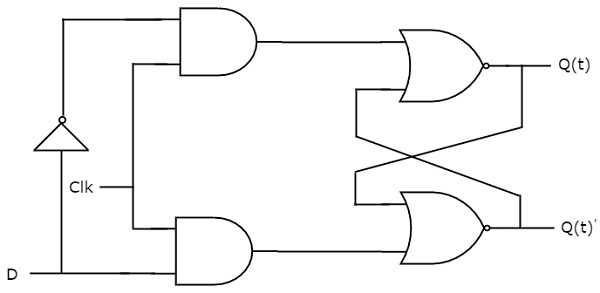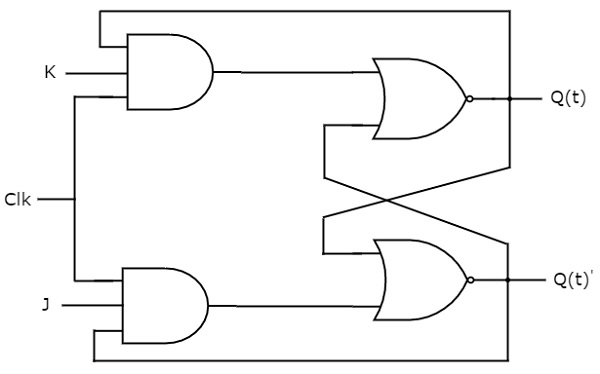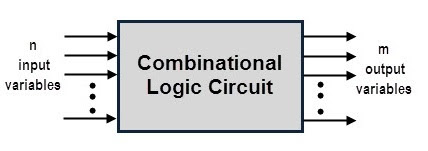### D Flip-Flop

• The D flip-flop can only work with positive or negative clock transitions. D latch, on the other hand, uses an enable signal. That is, except for active transition of the clock signal, the output of the D flip-flop is unaffected by changes in the input. The D flip-flop circuit diagram is given in the graphic below.• This circuit has a single D input and two Qt & Qt' outputs. D flip-flops operate similarly to D latches. However, this flip-flop only affects the outputs when the clock signal is applied in a positive transition rather than active enable.

• The following table shows the state table of D flip-flop.

 D Qt+1t+1 0 0 1 1

• As a result, D always flip-flops. Hold the data accessible on the data input, D of the earlier positive clock signal transition. The following state equation can be written directly from the prior state table:
Qt + 1 = D

• For every positive transition of the clock signal, the next state of the D flip-flop is always equal to data input, D. As a result, D flip-flops are suitable for use in registers, shift registers, and some counters.

JK Flip-Flop:

• The updated variant of the SR flip-flop is the JK flip-flop. It only works with positive or negative clock transitions. The JK flip-flop circuit diagram is given in the graphic below.• This circuit has two inputs J & K and two outputs Q t & Q t'. The operation of JK flip-flops is similar to that of SR flip-flops. Here,  the inputs of the SR flip-flops are considered as S = JQ t` and R = KQ t`, and the SR flip-flops modified to a combination of four inputs are used.

• The following table shows the state table of JK flip-flop.

 J K Qt + 1 0 0 Qt 0 1 0 1 0 1 1 1 Qt’

• Where Qt and Qt + 1 are the current state and the next state, respectively. Therefore, the JK flip-flop can be used for any of these four functions, such as hold, reset, set, and complement the current state based on the input conditions when the positive transition of the clock signal is applied. increase. The following table shows the characteristics table of JK flip-flops.

 Present Input Present State Next State J K Qt Qt+1 0 0 0 0 0 0 1 1 0 1 0 0 0 1 1 0 1 0 0 1 1 0 1 1 1 1 0 1 1 1 1 0
• As shown in the following figure, we use a three-variable KMap to get a simplified expression for the next state Qt + 1 and a three-variable KMap for the next state Qt + 1.• The maximum possible grouping of adjacent things is already shown in the figure. Therefore, the simplified expression for the next state is Qt + 1.
Q (t + 1) = JQ (t)' + K'Q (t)

## Introduction to Combinational Logic Circuits

• The digital system, which can be created with various logic gates, processes digital signals. These logic circuits are made up of various logic gates that are connected in certain ways to produce the desired output. Sequential logic circuits and combinational logic circuits are the two basic forms of digital logic circuits. Combinational logic circuits are briefly discussed in this article.

Combinational Logic Circuits

• A combinational logic circuit is one in which the output is determined by the current state of the logic inputs. Combination logic refers to the joining of two or more logic gates to create a needed function in which the output at any one time is solely determined by the input.

• A combinational circuit's core building pieces are logic gates. Multiplexers and demultiplexers, comparators, adders and subtractors, and other complex combinational circuits can be implemented using a combination of logic gates.

• Input variables, logic gates, and output variables make up a combinational circuit. The logic gates accept inputs and generate output signals based on the type of logic gate operation.

• This method transforms the binary data provided at the input to produce the needed output data. The schematic depiction of a generalised combinational logic circuit with n input variables and m output variables is shown below.• In the above figure, There will be 2n possible combinations of bits at the input because there are n input variables. Each output is expressed using a Boolean expression of input variables. As a result, m Boolean expressions can be used to express the result of the aforementioned generalised combinational logic circuit.

• The circuit in the diagram above accepts binary variables and generates outputs based on the logical combination of gates.

Design Procedure of Combinational Logic Circuits

A combinational circuit can be designed using the following steps.

• Number of available input variables and required output variables are identified and determined.

• Each and every input and output variable is represented by symbols (alphabets).

• The link between the input and output variables is expressed.

• The truth table is constructed to show the link between the input and output variables.

• In terms of input variables, obtain the Boolean expression for each output variable.

• Boolean expressions of various output variables are minimised.

• The logic diagram is created by implementing reduced Boolean expressions.

Various simplification strategies are available to reduce the number of gates in Boolean expressions, resulting in lower implementation costs. Boolean algebra theorems and identities, Karnaugh maps (K-maps), Quinne-McCluskey tabulation, and other techniques are among them. The following rules are often preferred for hardware implementation of the combinational circuit.

• The circuit should be implemented in such a way that it has the fewest possible gates and inputs.

• The number of linkages between gates should be kept to a bare minimum, and the propagation time should be as low as possible.

• The gates' driving capabilities should constantly be limited.

This method of designing a combinational circuit for a tiny circuit is straightforward, effective, and classic. The number of gates required increases as the circuit becomes more sophisticated, as does the number of wires between them. As a result, such circuits may be less dependable and time-consuming to design.

Most combinational circuits are available in integrated circuits (ICs), which are widely employed in the construction of digital systems, to address these issues. These ICs are divided as small, medium, big, and very large scale ICs based on gate integration capability.

In digital systems, medium scale integrated (MSI) ICs are used to execute specialised digital functions such as addition, multiplexing, de-multiplexing, encoding, decoding, comparison, and so on.